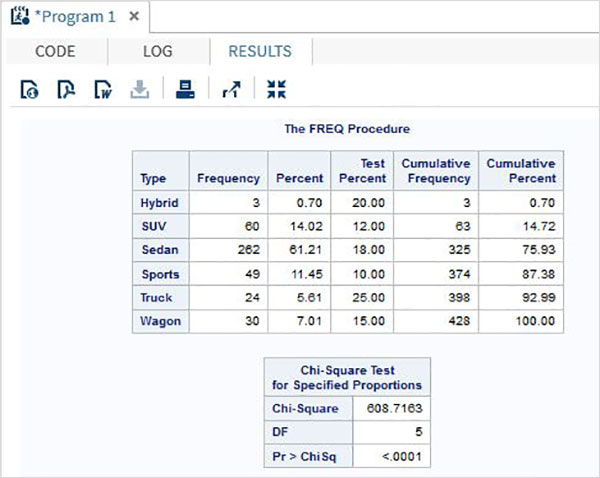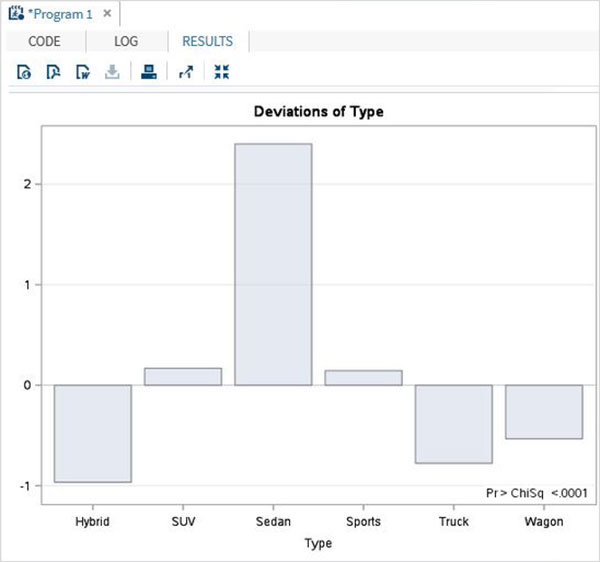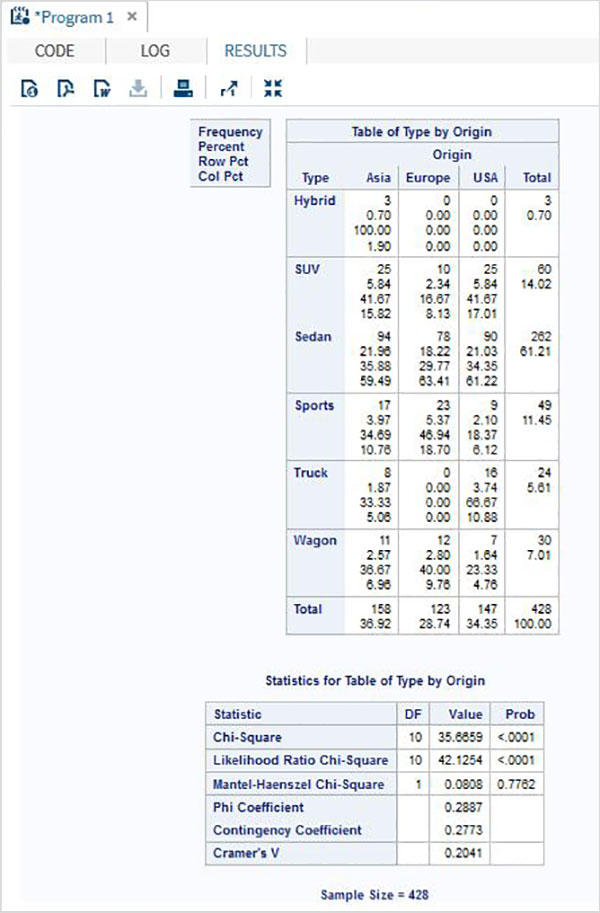# SAS - Chi Square

A chi-square test is used to examine the association between two categorical variables. It can be used to test both extent of dependence and extent of independence between Variables. SAS uses PROC FREQ along with the option chisq to determine the result of Chi-Square test.

### Syntax

The basic syntax for applying PROC FREQ for Chi-Square test in SAS is −

```PROC FREQ DATA = dataset;
TABLES variables
/CHISQ TESTP = (percentage values);
```

Following is the description of the parameters used −

• Dataset is the name of the dataset.

• Variables are the variable names of the dataset use in chi-square test.

• Percentage Values in the TESTP statement represent the percentage of levels of the variable.

### Example

In the below example we consider a chi-square test on the variable named type in the dataset SASHELP.CARS. This variable has six levels and we assign percentage to each level as per the design of the test.

```proc freq data = sashelp.cars;
tables type
/chisq
testp = (0.20 0.12 0.18 0.10 0.25 0.15);
run;
```

When the above code is executed, we get the following result −We also get the bar chart showing the deviation of the variable type as shown in the following screenshot.## Two Way chi-square

Two way Chi-Square test is used when we apply the tests to two variables of the dataset.

### Example

In the below example we apply chi-square test on two variables named type and origin. The result shows the tabular form of all combinations of these two variables.

```proc freq data = sashelp.cars;
tables type*origin
/chisq
;
run;
```

When the above code is executed, we get the following result −